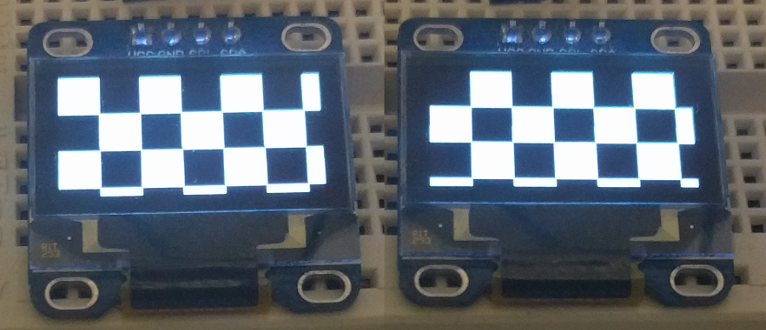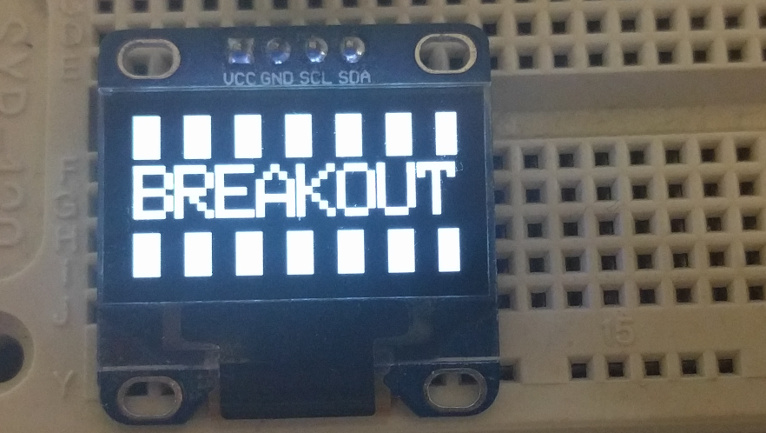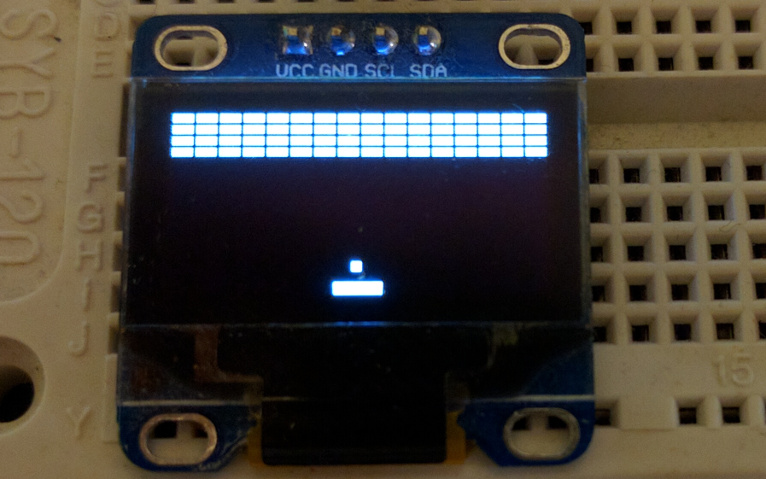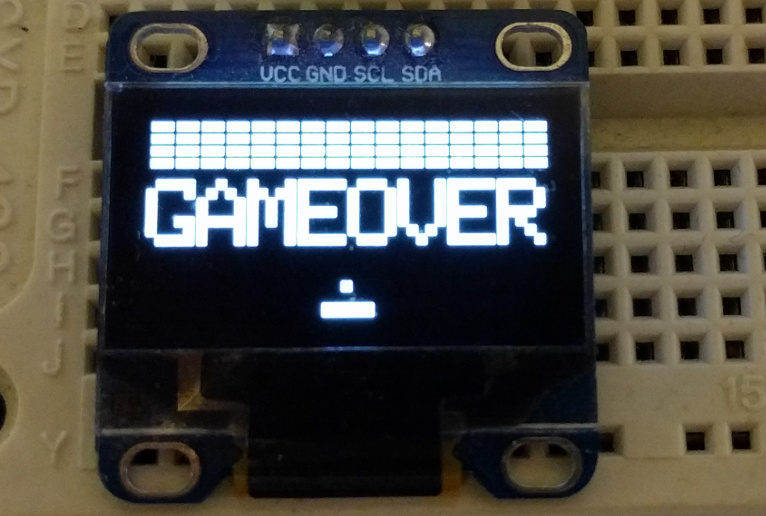I have pyboard, OLED display (SSD1306) and joystick (Keyes_SJoys), so I decided to try to make breakout clone. First of all I decided to create something like a little framework, that will be a bit similar to React, and all game can be formalized just in two functions:

• `(state) → new-state` – controller that updates state;
• `(state) → primitives` – view that converts state to generator of primitives.

For example, code that draws chess cells and invert it on click, will be like:

``````from lib.ssd1306 import Display
from lib.keyes import Joystick
from lib.engine import Game, rectangle, text

def is_filled(x, y, inverted):
fill = (x + y) % 40 == 0
if inverted:
return not fill
else:
return fill

def view(state):
# Display data available in state['display']
for x in range(0, state['display']['width'], 20):
for y in range(0, state['display']['height'], 20):
# rectangle is bundled view that yields points
yield from rectangle(x=x, y=y, w=20, h=20,
fill=is_filled(x, y, state['inverted']))

def controller(state):
# Joystick data available in state['display']
if state['joystick']['clicked']:
return dict(state, inverted=not state['inverted'])
else:
return state

initial_state = {'inverted': False}
chess_deck = Game(display=Display(pinout={'sda': 'Y10',
'scl': 'Y9'},
height=64,
external_vcc=False),
joystick=Joystick('X1', 'X2', 'X3'),
initial_state=initial_state,
view=view,
controller=controller)

if __name__ == '__main__':
chess_deck.run()
``````

In action:From the code you can see, that views can be easily nested with `yield from`. So if we want to move cell to separate view:

``````def cell(x, y, inverted):
yield from rectangle(x=x, y=y, w=20, h=20,
fill=is_filled(x, y, inverted))

def view(state):
for x in range(0, state['display']['width'], 20):
for y in range(0, state['display']['height'], 20):
yield from cell(x, y, state['inverted'])
``````

And another nice thing about this approach, is that because of generators we consume not a lot of memory, if we’ll make it eager, we’ll fail with `MemoryError: memory allocation failed` soon.

Back to breakout, let’s start with views, first of all implement splash screen:

``````def splash(w, h):
for n in range(0, w, 20):
yield from rectangle(x=n, y=0, w=10, h=h, fill=True)

yield from rectangle(x=0, y=17, w=w, h=30, fill=False)
# text is bundled view
yield from text(x=0, y=20, string='BREAKOUT', size=3)

def view(state):
yield from splash(state['display']['width'],
state['display']['height'])
``````

It will draw a nice splash screen:On splash screen game should be started when user press joystick, so we should update code a bit:

``````GAME_NOT_STARTED = 0
GAME_ACTIVE = 1
GAME_OVER = 2

def controller(state):
if state['status'] == GAME_NOT_STARTED and state['joystick']['clicked']:
state['status'] = GAME_ACTIVE
return state

initial_state = {'status': GAME_NOT_STARTED}
``````

Now when joystick is pressed, game changes `status` to `GAME_ACTIVE`. And now it’s time to create view for game screen:

``````BRICK_W = 8
BRICK_H = 4
BRICK_BORDER = 1
BRICK_ROWS = 4

BALL_W = 3
BALL_H = 3

def brick(data):
yield from rectangle(x=data['x'] + BRICK_BORDER,
y=data['y'] + BRICK_BORDER,
w=BRICK_W - BRICK_BORDER,
h=BRICK_H - BRICK_BORDER,
fill=True)

yield from rectangle(x=data['x'], y=data['y'],
fill=True)

def ball(data):
yield from rectangle(x=data['x'], y=data['y'],
w=BALL_W, h=BALL_H, fill=True)

def deck(state):
for brick_data in state['bricks']:
yield from brick(brick_data)

yield from ball(state['ball'])

def view(state):
if state['status'] == GAME_NOT_STARTED:
yield from splash(state['display']['width'],
state['display']['height'])
else:
yield from deck(state)

def get_initial_game_state(state):
state['status'] = GAME_ACTIVE
state['bricks'] = [{'x': x, 'y': yn * BRICK_H}
for x in range(0, state['display']['width'], BRICK_W)
for yn in range(BRICK_ROWS)]
state['ball'] = {'x': (state['display']['width'] - BALL_W) / 2,
'y': state['display']['height'] - PADDLE_H * 2 - BALL_W}
return state

def controller(state):
if state['status'] == GAME_NOT_STARTED and state['joystick']['clicked']:
state = get_initial_game_state(state)
return state
``````And last part of views – game over screen:

``````def game_over():
yield from text(x=0, y=20, string='GAMEOVER', size=3)

def view(state):
if state['status'] == GAME_NOT_STARTED:
yield from splash(state['display']['width'],
state['display']['height'])
else:
yield from deck(state)
if state['status'] == GAME_OVER:
yield from game_over()
``````So we ended up with views, now we should add ability to move paddle with joystick:

``````def update_paddle(paddle, joystick, w):

def controller(state):
if state['status'] == GAME_NOT_STARTED and state['joystick']['clicked']:
state = get_initial_game_state(state)
elif state['status'] == GAME_ACTIVE:
state['display']['width'])
return state
``````

Never mind performance, it’ll be fixed in the end of the article:

Now it’s time for the hardest thing – moving and bouncing ball, so there’s no real physics, for simplification ball movements will be represented as `vx` and `vy`, so when ball:

• initialised: `vx = rand(SPEED)`, `vy = √SPEED^2 - vx^2`;
• hits the top wall: `vy = -vy`;
• hits the left or right wall: `vx = -vx`:
• hits the brick or paddle: `vx = vx + (0.5 - intersection) * SPEED`, where intersection is between `0` and `1`; `vy = √SPEED^2 - vx^2`.

And I implemented something like this with a few hacks:

``````BALL_SPEED = 6
BALL_SPEED_BORDER = 0.5

def get_initial_game_state(state):
state['status'] = GAME_ACTIVE
state['bricks'] = [{'x': x, 'y': yn * BRICK_H}
for x in range(0, state['display']['width'], BRICK_W)
for yn in range(BRICK_ROWS)]

# Initial velocity for ball:
ball_vx = BALL_SPEED_BORDER + pyb.rng() % (BALL_SPEED - BALL_SPEED_BORDER)
ball_vy = -math.sqrt(BALL_SPEED ** 2 - ball_vx ** 2)
state['ball'] = {'x': (state['display']['width'] - BALL_W) / 2,
'y': state['display']['height'] - PADDLE_H * 2 - BALL_W,
'vx': ball_vx,
'vy': ball_vy}
return state

def calculate_velocity(ball, item_x, item_w):
"""Calculates velocity for collision."""
intersection = (item_x + item_w - ball['x']) / item_w
vx = ball['vx'] + BALL_SPEED * (0.5 - intersection)
if vx > BALL_SPEED - BALL_SPEED_BORDER:
vx = BALL_SPEED - BALL_SPEED_BORDER
elif vx < BALL_SPEED_BORDER - BALL_SPEED:
vx = BALL_SPEED_BORDER - BALL_SPEED

vy = math.sqrt(BALL_SPEED ** 2 - vx ** 2)
if ball['vy'] > 0:
vy = - vy
return vx, vy

def collide(ball, item, item_w, item_h):
return item['x'] - BALL_W < ball['x'] < item['x'] + item_w \
and item['y'] - BALL_H < ball['y'] < item['y'] + item_h

def update_ball(state):
state['ball']['x'] += state['ball']['vx']
state['ball']['y'] += state['ball']['vy']

# Collide with left/right wall
if state['ball']['x'] <= 0 or state['ball']['x'] >= state['display']['width']:
state['ball']['vx'] = - state['ball']['vx']

# Collide with top wall
if state['ball']['y'] <= 0:
state['ball']['vy'] = -state['ball']['vy']

state['ball'].update(vx=vx, vy=vy)

# Collide with brick
for n, brick in enumerate(state['bricks']):
if collide(state['ball'], brick, BRICK_W, BRICK_H):
vx, vy = calculate_velocity(state['ball'], brick['x'], BRICK_W)
state['ball'].update(vx=vx, vy=vy)
state['bricks'].pop(n)

return state

def controller(state):
if state['status'] == GAME_NOT_STARTED and state['joystick']['clicked']:
state = get_initial_game_state(state)
elif state['status'] == GAME_ACTIVE:
state['display']['width'])
state = update_ball(state)
return state
``````

And it seems to be wroking:

So now the last part, we should show “Game Over” when ball hits the bottom wall or when all bricks destroyed, and then start game again if user clicks joystick:

``````def is_game_over(state):
return not state['bricks'] or state['ball']['y'] > state['display']['height']

def controller(state):
if state['status'] in (GAME_NOT_STARTED, GAME_OVER)\
and state['joystick']['clicked']:
state = get_initial_game_state(state)
elif state['status'] == GAME_ACTIVE:
state['display']['width'])
state = update_ball(state)
if is_game_over(state):
state['status'] = GAME_OVER
return state
``````

And it works too:

Performance so bad because drawing pixel on the screen is relatively time consuming operation, and we can easily fix performance by just decreasing count of bricks:

``````BRICK_W = 12
BRICK_H = 6
BRICK_BORDER = 4
BRICK_ROWS = 3
``````

And now it’s smooth:

Source code.